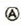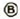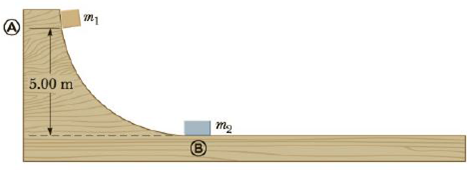Chapter 6, Problem 62AP

Chapter
Section
Textbook Problem

Consider a frictionless track as shown in Figure P6.62. A block of mass m1 = 5.00 kg is released from. It makes a head-on elastic collision atwith a block of mass m2= 10.0 kg that is initially at rest. Calculate the maximum height to which m1 rises after the collision.Figure P6.62

To determine
The height to which m1 rises after collision.

Explanation

Given Info:

Mass of the block at position A is 5.00kg , the mass of the block at B is 10.0kg , initial speed of mass at position B is zero.

According to conservation of energy , kinetic energy will be equal to the potential energy of the mass.

Formula to calculate the kinetic energy of the mass 1 is at position A is ,

KE=12m1v12 (I)

• m1 is the block released from top.
• v1 is the speed of the mass 1 at position A before collision

Formula to calculate the potential energy of the mass 1 at position A is,

PE=m1gh (II)

• g is the acceleration due to gravity
• h is the height of the mass m1

According to the principle of conservation of energy, Potential energy of mass 1 at position A is converted into its equivalent the kinetic energy at position B.

Equate (I) and (II) to calculate v1 .

12m1v12=m1ghv12=2ghv1=2gh

Substitute 9.80ms2 for g and 5.00m for h in the above equation to calculate v1 .

v1=2(9.80ms2)(5.00m)=98.0m2s2=9.899ms19.90ms1

Collisions conserve momentum.

Apply conservation of momentum before and after collision for the masses m1 and m2

m1v1+m2v2=m1v1+m2v2 (III)

• v2 is the speed of the mass m2 at position B
• v1 is the speed of mass m1 after collision
• v2 is the speed of mass m2 after collision
• m2 is the mass of the mass of the block at rest at bottom

Initial speed of mass2 is zero.

Substitute 0m/s for v2 in the above equation and rewrite in terms of v2 .

m1v1+m2(0m/s)=m1v1+m2v2m2v2=m1v1m1v1=m1(v1v1)v2=m1m2(v1v1)

But for elastic collision

v1v2=(v1v2)

Since initial speed of m2 is zero,

Substitute 0m/s for v2 in the above equation and rewrite in terms of v2 .

v2=v1+v1 (IV)

Substitute the above equation (IV) in (III) to calculate v1

Still sussing out bartleby?

Check out a sample textbook solution.

See a sample solution

The Solution to Your Study Problems

Bartleby provides explanations to thousands of textbook problems written by our experts, many with advanced degrees!

Get Started

Vitamin C deficiency symptoms include: red spots. loose teeth. anemia. all of the above.

Nutrition: Concepts and Controversies - Standalone book (MindTap Course List)

What does the word apparent mean in apparent visual magnitude?

Horizons: Exploring the Universe (MindTap Course List)

What is hereditarianism, and what is the invalid assumption it makes?

Human Heredity: Principles and Issues (MindTap Course List)

Lignin is not found in stems of_____. a. mosses b. ferns c. monocots d. a and b

Biology: The Unity and Diversity of Life (MindTap Course List)